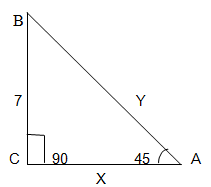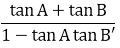# RD Sharma Class 10 Ex 5.2 Solutions Chapter 5 Trigonometric Ratios

In this chapter, we provide RD Sharma Class 10 Ex 5.2 Solutions Chapter 5 Trigonometric Ratios for English medium students, Which will very helpful for every student in their exams. Students can download the latest RD Sharma Class 10 Ex 5.2 Solutions Chapter 5 Trigonometric Ratios pdf, Now you will get step by step solution to each question.

# Chapter 5: Trigonometric Ratios Exercise – 5.2

### Question: 1

sin 45° sin 30° + cos 45° cos 30°

### Solution:

Sin 45°sin 30° + cos 45° cos 30°      

We know that by trigonometric ratios we have,

Substituting the values in equation 1, we get

### Question: 2

sin 60° cos 30° + cos 60° sin 30°

### Solution:

sin 60° cos 30° + cos 60° sin 30° 

By trigonometric ratios we have,

Substituting the values in equation 1, we get

### Question: 3

cos 60° cos 45° – sin 60° sin 45°

### Solution:

cos 60° cos 45° – sin 60° sin 45°  

We know that by trigonometric ratios we have,

Substituting the values in equation 1, we get

### Question: 4

sin230° + sin245° + sin260° + sin290°

### Solution:

sin230° + sin245° + sin260° + sin290°   

We know that by trigonometric ratios we have,

Substituting the values in equation 1, we get

### Question: 5

cos230° + cos245° + cos260° + cos290°

### Solution:

cos230° + cos245° + cos260° + cos290° 

We know that by trigonometric ratios we have,

Substituting the values in equation 1, we get

### Question: 6

tan230° + tan245° + tan260°

### Solution:

tan230° + tan245° + tan260°          

We know that by trigonometric ratios we have,

Substituting the values in equation 1, we get

### Question: 7

2 sin230° − 3 cos245° + tan260°

### Solution:

2sin230° − 3cos245° + tan260°         

We know that by trigonometric ratios we have,

Substituting the values in equation 1, we get

### Question: 8

sin30° cos245°+ 4 tan230°+ 1/2sin2 90° – 2 cos2 90°+ 1/24 cos2

### Solution:

sin30° cos245°+ 4 tan230°+ 1/2sin2 90° – 2 cos2 90°+ 1/24 cos20° 

We know that by trigonometric ratios we have,

Substituting the values in equation 1, we get

### Question: 9

4(sin460° + cos430°) − 3(tan260° − tan245°) + 5cos245°

### Solution:

4(sin460° + cos430°) − 3(tan260° − tan245°) + 5cos245°   

We know that by trigonometric ratios we have,

Substituting the values in equation 1, we get

Question: 10

(cosec45° sec30°) (sin230° + 4 cot245° − sec60°)

### Solution:

(cosec245° sec230°)(sin230° + 4 cot245° − sec260°)      

We know that by trigonometric ratios we have,

Substituting the values in equation 1, we get

### Question: 11

cosec30°cos 60° tan45° sin90° sec45°cot 30°

### Solution:

= cosec30°cos 60° tan45° sin90° sec45° cot 30°

### Question: 12

cot230° − 2cos260° − 3/4 sec245° – 4 sec230°

### Solution:

= cot230° − 2cos260° − 3/4 sec245° – 4 sec230°

### Question: 13

(cos 0° + sin 45° + sin 30°) (sin 90 − cos 45° + cos 60°)

### Solution:

Given, (cos 0° + sin 45° + sin 30°) (sin 90 − cos 45° + cos 60°)

Given,

Given,

### Question: 16

4(sin430° + cos260°) − 3(cos245° − sin290°) − sin260°

### Solution:

Given,

4(sin430° + cos260°) − 3(cos245° − sin290°) − sin260°

Given,

= 3 + 2 + 4

= 9

Given,

2 sin 3x = √3

### Solution:

Given,

2 sin 3x = √3

⟹ sin 3x = √3/2

⟹ sin 3x = sin 60°

⟹ 3x = 60°

⟹ x = 20°

x/2 = 30°

x = 60°

√3 sin x = cos x

### Solution:

√3 tan x = 1

tan x = 1/√3

∴ tan x =  tan45°

x = 45°

### Question: 23

tan x = sin 45° cos 45° + sin 30°

tan x = 1

tan x = 45°

x = 45°

### Question: 24

√3 tan 2x = cos 60°+ sin 45°cos 45°

2x = 30°

x = 15°

### Question: 25

cos 2x = cos 60° cos 30° + sin 60° sin 30°

2x = 30°

x = 15°

### Question: 26

If θ = 30°, verify

(iv) cos 3θ = 4cos3θ − 3cos θ cos 3θ = 4 cosθ – 3 cos θ

### Solution:

Substitute θ = 30° θ = 30° in equation (i)

Therefore, LHS = RHS

Substitute θ = 30° θ = 30°

Therefore, LHS = RHS.

Substitute θ = 30°θ = 30°

Therefore, LHS = RHS

(iv) cos 3θ = 4cos3θ − 3cos θ cos 3θ = 4 cos3θ – 3 cos θ

Solution:

LHS = cos 3θ

Substitute θ = 30°

= cos 3(30°) = cos 90°

= 0

RHS = 4 cos3θ – 3 cos θ

= 4 cos330° − 3 cos 30°

= 0

Therefore, LHS = RHS.

### Question: 27

If A = B = 60°. Verify

(i) cos (A – B) = cos A cos B + sin A sin B

### Solution:

cos (A – B) = cos A cos B + sin A sin B … (i)

Substitute A and B in (i)

⟹ cos (60° – 60°) = cos 60° cos 60° + sin 60° sin 60°

⟹ 1 = 1

Therefore, LHS = RHS

(ii) Substitute A and B in (i)

⟹ sin (60° – 60°) = sin 60° cos 60° – cos 60° sin 60°

⟹ sin0° = 0

⟹ 0 = 0

Therefore, LHS = RHS

A = 60°, B = 60° we get,

tan 0° = 0

0 = 0

Therefore, LHS = RHS

### Question: 28

If A = 30°, B = 60° verify:

(i) Sin (A + B) = Sin A cos B + Cos A Sin B

(ii) Cos (A + B) = Cos A Cos B – Sin A Sin B

### Solution:

(i) A = 30°, B = 60° we get

Sin (30° + 60°) = Sin 30° Cos 60° + Cos 30°  Sin 60°

Sin (90°) = 1 ⟹ 1 = 1

Therefore, LHS = RHS

(ii) Cos (A + B) = Cos A Cos B – Sin A Sin B

A = 30°, B = 60° we get

Cos (30° + 60°) = Cos 30° Cos 60° – Sin 30° Sin 60°

0 = 0

Therefore, LHS = RHS

### Question: 29

If sin(A + B) = 1 and cos(A – B) = 1, 0° < A + B ≤ 90°, A ≥ B find A and B.

### Solution:

Given,

sin(A + B) = 1 this can be written as sin (A + B) = sin (90°) sin (90°)

cos(A – B) = 1 this can be written as cos (A – B) = cos (0°) cos (0°)

⟹ A + B = 90°

A – B = 0°

2A = 90°

A = 90°/2

A = 45°

Substitute A value in A – B = 0°

45°– B = 0°

B = 45°

Hence, the value of A = 45°and B = 45°

### Question: 30

If tan (A – B) = 1/√3 and tan (A + B) = √3, 0°<A + B ≤ 90°, A > B find A and B

### Solution:

Given,

A – B = 30°   … 1

A + B = 60° … 2

Solve equations 1 and 2

A + B = 30°

A – B = 60°

2A = 90°

A = 90°/2

A = 45°

Substitute the value of A in equation 1

45°+ B = 30°

B = 30° – 45°

B = 15°

The value of A = 45°and B = 15°

### Question: 31

If sin (A – B) = 1/2 and cos (A + B) = 1/2, 0°<A + B ≤ 90°, A < B. find A and B.

### Solution:

Given,

A + B = 60° … 2

Solve equations 1 and 2

A + B = 60°

A – B = 30°

2A  = 90°

A = 90°/2

A = 45°

Substitute the value of A in equation 2

45°+ B = 60°

B = 60° – 45°

B = 15°

The value of A = 45°and B = 15°

### Question: 32

In a Δ ABC right angled triangle at B, ∠A = ∠C. Find the values of:

1. sin A cos C + cos A sin C

2. sin A sin B + cos A cos B

### Solution:

1. since, it is given as ∠A = ∠C

the value of A and C is 45°, the value of angle  B is 90°

because the sum of angles of triangle is 180°

⟹ sin 45° cos 45° + cos 45° sin 45°

⟹ 1

The value of sin A cos C + cos A sin C is 1

2. since, it is given as  ∠A = ∠C

the value of A and C is 45°, the value of angle  B is 90°

because the sum of angles of triangle is 180°

⟹ sin 45°sin 90° + cos 45°sin 90°

The value of sin A sin B + cos A cos B is 1/√2

### Question: 33

Find the acute angle A and B, if sin (A + 2B) = √3/2 and cos(A + 4B) = 0, A > B.

### Solution:

Given,

A + 2B = 60° … 1

Cos (A + 4B) = 0

A + 4B = sin−1(90) sin−1(90)

A + 4B = 90°    … 2

Solve equations 1 and 2

2B = 30°

B = 30°/2

B = 15°

Substitute B value in equation 2

A + 4B = 90°

A + 4(15°) = 90°

A + 60° = 90°

A = 90° – 60°

A = 30°

The value of A = 30° and B = 15°

### Question: 34

In ΔPQR, right angled at Q, PQ = 3 cm and PR = 6 cm. Determine ∠ P and ∠ R.

### Solution:

In ΔPQR, right angled at Q, PQ = 3 cm and PR = 6 cm

By Pythagoras theorem,

PR= PQ2 + QR2

⟹ 62 = 32 + QR2

⟹ QR2 = 36 – 9

⟹ QR = √27

⟹ QR = 3√3

sin R = 3/6 = 1/2 = sin30°

∠R = 30°

As we know, Sum of angles in a triangle = 180

∠P + ∠Q + ∠R = 180°

⟹ ∠P + 90° + 30° = 180°

⟹ ∠P = 180° – 120°

⟹ ∠P = 60°

Therefore, ∠R = 30°

And, ∠P = 60°

### Question: 35

If sin (A – B) = sin A cos B – cos A sin B and cos (A – B) = cos A cos B + sin A sin B, find the values of sin 15 and cos 15.

### Solution:

Given,

sin (A – B) = sin A cos B – cos A sin B

And, cos (A – B) = cos A cos B + sin A sin B

We need to find, sin 15 and cos 15.

Let A = 45 and B = 30

sin 15 = sin (45- 30) = sin 45 cos 30 – cos 45 sin 30

cos 15 = cos (45- 30) = cos 45 cos 30 – sin 45 sin 30

### Question: 36

In a right triangle ABC, right angled at C, if ∠B = 60° and AB = 15 units. Find the remaining angles and sides.

Sin 60°= x/15

cos 60°= x/15

x = 7.5 units

### Question: 37

In ΔABC is a right triangle such that ∠C = 90°, ∠A = 45°and BC = 7 units. Find the remaining angles and sides.

### Solution:Here, ∠C = 90° and ∠A = 45°

We know that,

∠A + ∠B + ∠C = 180°

⟹ 45°+ 90° + ∠C = 180°

⟹ 135° + ∠C = 180°

⟹ ∠C = 180° – 135°

⟹ ∠C = 45°

The value of the remaining angle C is 45°

Now, we need to find the sides x and y here,

y =7√2 units

x = 7 units

the value of x = 7 units and y = √2 units

### Question: 38

In a rectangle ABCD, AB = 20 cm, ∠BAC = 60°, calculate side BC and diagonals AC and BD.

### Solution:

Let AC = x cm and CB = y cm

⇒ x = 40 cm = AC

Similarly BD = 40 cm

Now,

### Question: 39

If A & B are acute angles such that tan A = 1/2 tan B = 1/3 and tan (A + B) =find A+B.

### Solution:

(A + B) = Tan-1 (1)

(A + B) = 45°

### Question: 40

Prove that: (√3 -1) (3 – cot 30°) = tan360° – 2 sin 60°

### Solution:

R.H.S ⟹ tan60° – 2 sin 60°

L.H.S = R.H.S

Hence prove

All Chapter RD Sharma Solutions For Class10 Maths

I think you got complete solutions for this chapter. If You have any queries regarding this chapter, please comment on the below section our subject teacher will answer you. We tried our best to give complete solutions so you got good marks in your exam.